# Free Ncert Class 6 Math Understanding Elementary Shapes Exercise 5.8

Understanding Elementary Shapes Class 6 Ex. 5.8

New Ncert Class 6 Math Free Solution.

Exercise 5.8

Question 1 :- Examine whether the following are polygons. If any one among them is not, say why?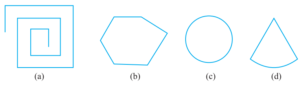Solution 1:-
(a) The given figure is not a closed figure. Therefore, it is not a polygon.
(b) The given figure is a polygon of six sides.
(c) The given figure is not a polygon because they are not made of line segments.
(d) The given figure is not a polygon because they are not made of line segments.

Question 2 :- Name the polygon.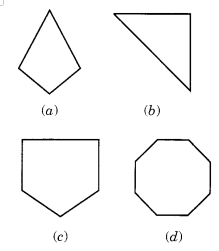Make two more examples of each of these.

Solution 2:-

Examples are :-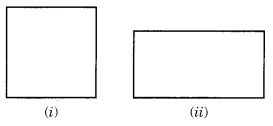(b) A Triangle.

Examples are :-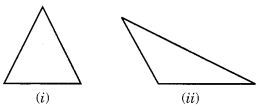(c) A Pantagon (5-sided).

Examples are :-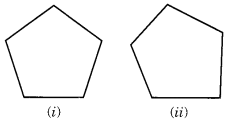(d) A Octagon.

Examples are :-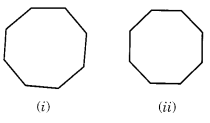Question 3 :- Draw rough sketch of a regular-hexagon. Connecting any three of its verticess, draw a triangle. Identify the type of the triangle you have drawn;.

Solution 3:-
A rough drawing of a regular hexagon is ABCDEF. If we join any three vertices like D, A and B, we get a scalene triangle DAB.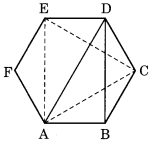But if we join the alternate vertices, we get an equilateral triangle EAC.

Question 4 :- Draw, a rough sketch of a regular-octagon. (Using squared paper if you wish). Draw a rectangle by joining exactly four of the vertices of the octagon;.

Solution 4:-
ABCDEFGH is a rough sketch of regular octagon. The rectangle known as GHCD is created by connecting the four vertices of the specified octagon.
.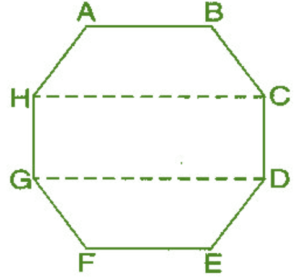Question 5 :- A diagonal is a line-segment that joins any two-vertices of the polygon and is not a side of the polygon. Draw a rough sketch of a pentagon and draw its all diagonals.

Solution 5:-
The rough drawing of a pentagon is ABCDE.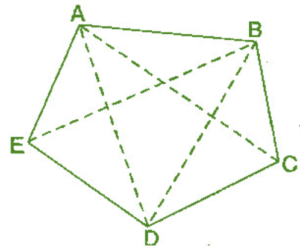The following diagonals can be obtained by linking any two of its vertices.